# Dynamic Discount Value Builder

The dynamic discount value builder defines how the discount value is calculated.

## Dynamic Discount Value Builder

Instead of using a static value for a given discount, you can also create formulas with mathematical and logical operators and you can assign a dynamic discount value. Discounts will be calculated during redemption based on the provided formula and metadata values.

The formula builder requires a static fallback value in case the dynamic discount value cannot be calculated from the given parameters in the formula.

You can either use the Formula builder utility with step-by-step dialogs that you fill out to build the formula from element building blocks or the Expression output with operators and operands.

The dynamic discount value applies to the following discount types:

• Amount
• Percentage
• Fixed amount
• Unit

and can be used when creating coupon campaigns, in-cart promotions, and referral codes.

## Context Specific

The operators and operands in the dynamic discount value builder depend on the context of the formula, such as the discount effect and other factors. Some of the operators and operands will not be available based on the specific configuration.

## Formula Builder

You can use the Formula builder utility with step-by-step dialogs to build the formula from element building blocks.

First, click the Formula button next to the discount value.

Then, begin to build your formula using the formula builder by choosing a type of operand followed by an operator. Click the X button to delete a number or expression and the + button to add a number or expression.

To remove an operator you have entered, click on it and click the Remove button.

To review your expression, choose Expression output

Click Save once you have finished defining your dynamic discount formula.

To edit your existing formula, click the Formula button next to the discount value.

To remove a saved formula, click — button next to the Formula button.

## Expression Output

You can view and edit the dynamic discount formula through a mathematical expression using operands and operators. Click  Save once you have finished defining your dynamic discount formula.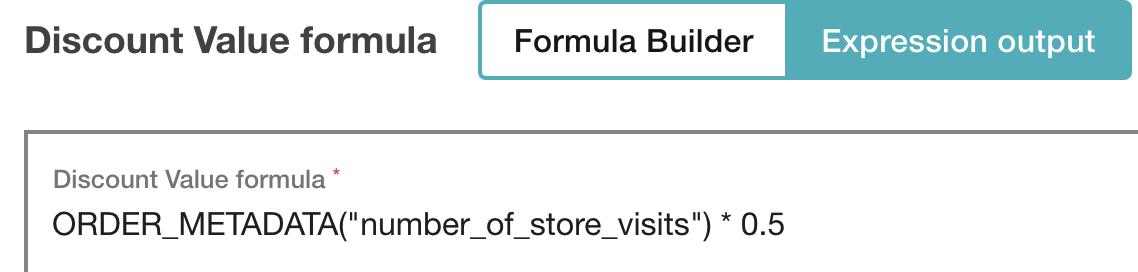## Operand Reference

You can see the discount values that you can create using different discount operands in the following table.

 Operand Definition Example Number Value is a Number. 2 Text Value is a String. Unique string of alphanumeric characters. Order metadata Value is an order metadata attribute of type Number or String.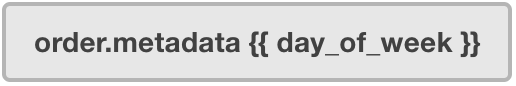`ORDER_METADATA("day_of_week")` Voucher metadata Value is a voucher metadata attribute of type  Number or String.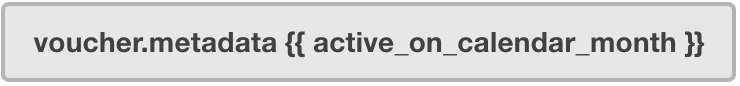`REDEEMABLE_METADATA("active_on_calendar_month")` Customer metadata Value is a customer metadata attribute of type Number or String.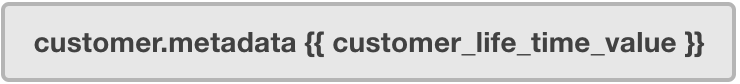`CUSTOMER_METADATA("customer_life_time_value")` Redemption metadata Value is a redemption metadata attribute of type Number or String.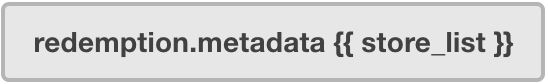`REDEMPTION_METADATA("store_list")` Publication metadata Value is a publication metadata attribute of type Number or String.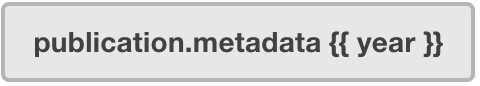`PUBLICATION_METADATA("year")` Brackets Groups expression elements together; i.e. adds parentheses to your formula.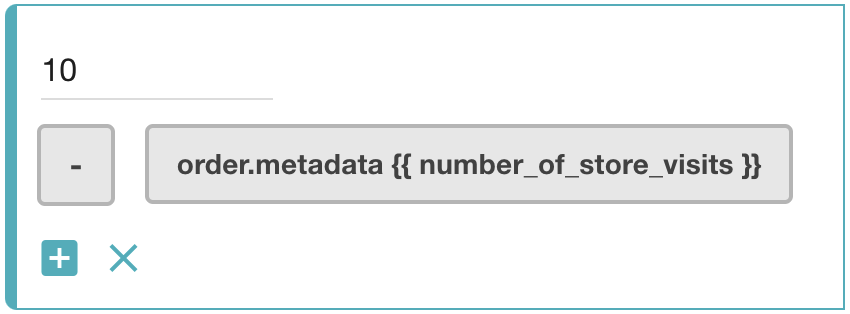`(10 - ORDER_METADATA("number_of_store_visits"))` Min Lowest value in a range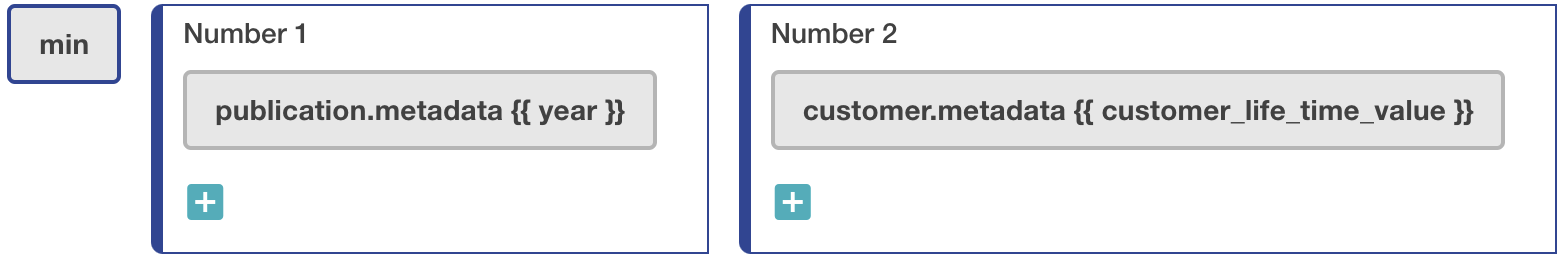`MIN(PUBLICATION_METADATA("year"); CUSTOMER_METADATA("customer_life_time_value"))` Max Highest value in a range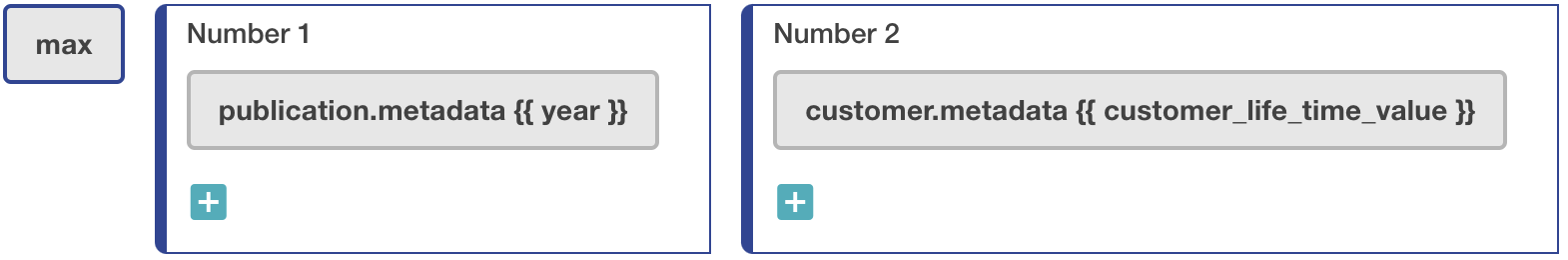`MAX(PUBLICATION_METADATA("year"); CUSTOMER_METADATA("customer_life_time_value"))` Power A number raised to a power; defines the base and exponent.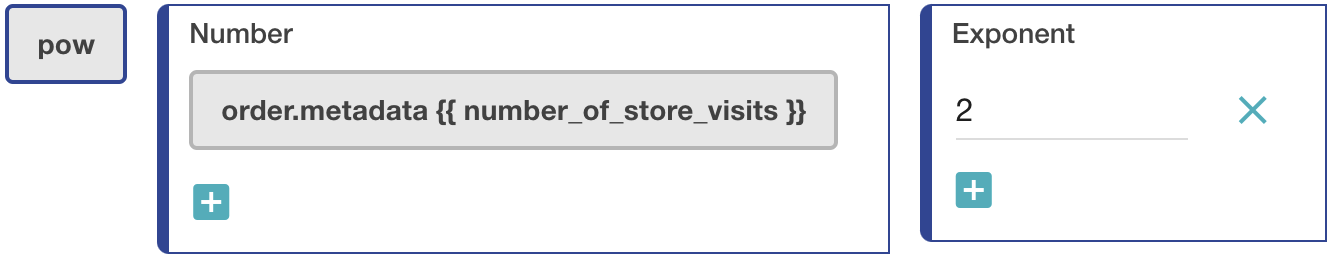`POW(ORDER_METADATA("number_of_store_visits");2)` Round Round a number to a specified number of digits. Positive precision refers to a decimal place indicator. Negative precision indicates an integer place indicator. Assume the customer lifetime value is 75.55. `ROUND(CUSTOMER_METADATA("customer_life_time_value");1)`  = 75.60 (precision value equal to 1) `ROUND(CUSTOMER_METADATA("customer_life_time_value");0)`  = 76.00 (precision value equal to 0) `ROUND(CUSTOMER_METADATA("customer_life_time_value");-1)`  = 80.00 (precision value equal to -1) Floor Round down to a specified number of digits. Positive precision refers to a decimal place indicator. Negative precision indicates an integer place indicator. Assume the customer lifetime value is 75.55. `FLOOR(CUSTOMER_METADATA("customer_life_time_value");1)`  = 75.50 (precision value equal to 1) `FLOOR(CUSTOMER_METADATA("customer_life_time_value");0)`  = 75.00 (precision value equal to 0) `FLOOR(CUSTOMER_METADATA("customer_life_time_value");-1)`  = 70.00 (precision value equal to -1) Ceil Round up to a specified number of digits. Positive precision refers to a decimal place indicator. Negative precision indicates an integer place indicator. Assume the customer lifetime value is 74.44. `CEIL(CUSTOMER_METADATA("customer_life_time_value");1)`  = 74.50 (precision value equal to 1) `CEIL(CUSTOMER_METADATA("customer_life_time_value");0)`  = 75.00 (precision value equal to 0) `CEIL(CUSTOMER_METADATA("customer_life_time_value");-1)`  = 80.00 (precision value equal to -1) If Value based on a logical expression; defines the logical test value, value if expression is true and value if expression is false.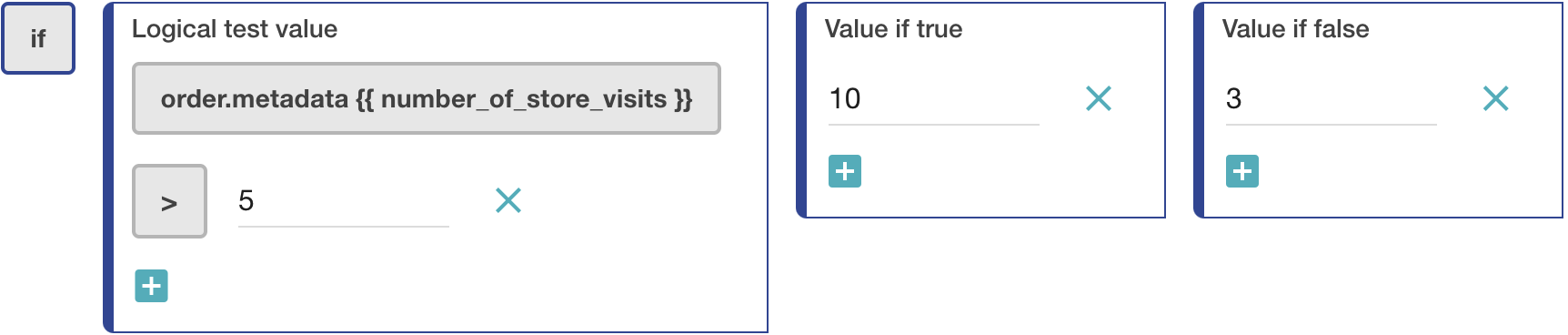`IF(ORDER_METADATA("number_of_store_visits") > 5;10;3)` Default to Returns the first non-null value of range.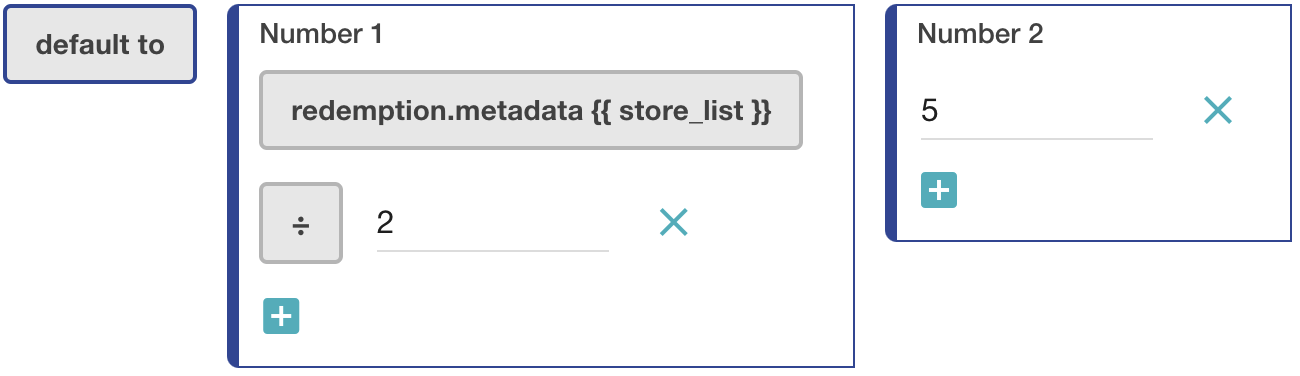`DEFAULT_TO(REDEMPTION_METADATA("store_list") / 2;5)` Order item price Returns order item price. For each item you can get a particular discount, e.g. (order item price) × 0.01 + 1. If the item price is 6.00, the discount is 1.06; if the item price is 10.00, the discount is 1.10.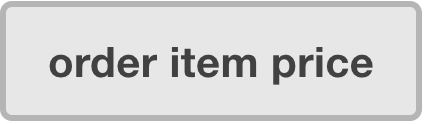`ORDER_ITEM_PRICE` Order item amount Returns order item amount; for stacked discounts, returns the original amount without any modifications due to other discounts.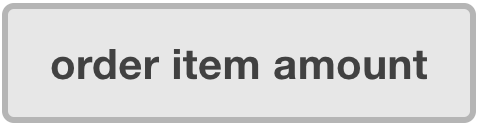`ORDER_ITEM_AMOUNT` Order item subtotal Returns order item subtotal; for stacked discounts, each additional discount is based on the result obtained after calculating the previous discount.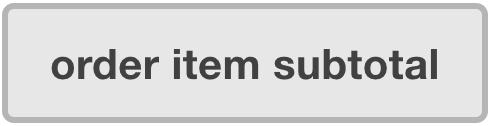`ORDER_ITEM_SUBTOTAL` Order item units quantity Returns order item quantity.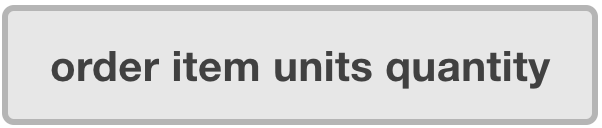`ORDER_ITEM_UNITS_QUANTITY` Order item metadata Returns order item metadata.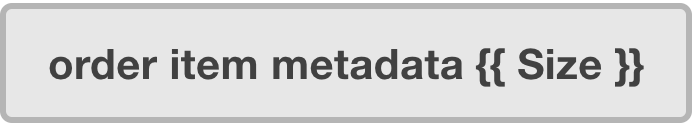`ORDER_ITEM_METADATA("Size")` Order item product metadata Returns order item product metadata.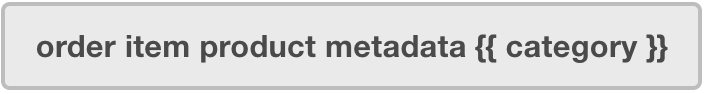`ORDER_ITEM_PRODUCT_METADATA("category")` Cheapest order item price Returns the price of the cheapest line item in the order. Read more.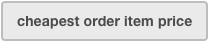`CHEAPEST_ORDER_ITEM_PRICE` Cheapest order item amount Returns the amount of the cheapest line items in the order; for stacked discounts, returns the original amount without any modifications due to other discounts. Read more.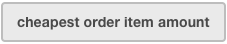`CHEAPEST_ORDER_ITEM_AMOUNT` Cheapest order item subtotal Returns the subtotal of the cheapest line items in the order; for stacked discounts, each additional discount is based on the result obtained after calculating the previous discount. Read more.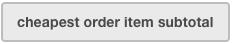`CHEAPEST_ORDER_ITEM_SUBTOTAL` Cheapest order item units quantity Returns total units of the cheapest order line items. Read more.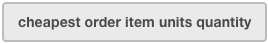`CHEAPEST_ORDER_ITEM_UNITS_QUANTITY` Cheapest order item metadata Returns the cheapest order item metadata of type Number or String. Read more.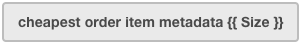`CHEAPEST_ORDER_ITEM_METADATA("Size")` Cheapest order item product metadata Returns the cheapest order item product metadata. Read more.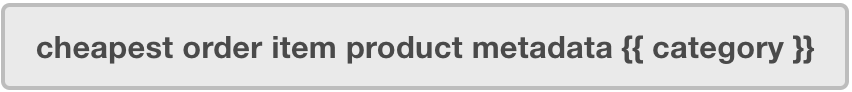`CHEAPEST_ORDER_ITEM_PRODUCT_METADATA("category")` Most expensive order item price Returns the price of the most expensive line item in the order. Read more.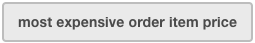`MOST_EXPENSIVE_ORDER_ITEM_PRICE` Most expensive order item amount Returns the amount of the most expensive line items in the order; for stacked discounts, returns the original amount without any modifications due to discounts. Read more.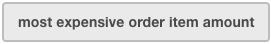`MOST_EXPENSIVE_ORDER_ITEM_AMOUNT` Most expensive order item subtotal Returns the subtotal of the most expensive line items in the order; for stacked discounts, each additional discount is based on the result obtained after calculating the previous discount. Read more.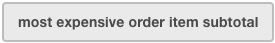`MOST_EXPENSIVE_ORDER_ITEM_SUBTOTAL` Most expensive order item units quantity Returns the total units of the most expensive order line items. Read more.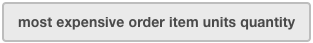`MOST_EXPENSIVE_ORDER_ITEM_UNITS_QUANTITY` Most expensive order item metadata Returns the most expensive order item metadata of type Number or String. Read more.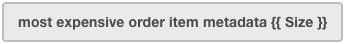`MOST_EXPENSIVE_ORDER_ITEM_METADATA("Size")` Most expensive order item product metadata Returns the most expensive order item product metadata. Read more.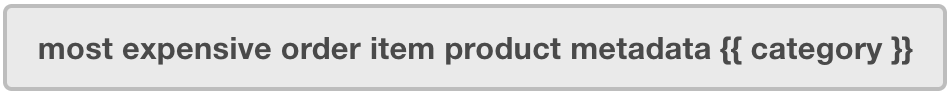`MOST_EXPENSIVE_ORDER_ITEM_PRODUCT_METADATA("category")` Order amount Returns the order total value, whole cart value.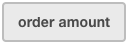`ORDER_AMOUNT` Order items quantity Returns the item count.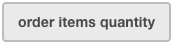`ORDER_ITEMS_QUANTITY` Order units quantity Returns the sum of the units ordered.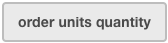`ORDER_UNITS_QUANTITY` Voucher redemption quantity Returns the voucher's redemption limit.`VOUCHER_REDEMPTION_QUANTITY` Voucher redeemed quantity Returns the number of times the voucher was redeemed.`VOUCHER_REDEEMED_QUANTITY` Voucher published count Returns the number of the times the voucher was published. This value will be either 0 or 1, so this parameter can be used as a security check. For example, if the voucher is assigned (published), then the customer gets a discount, otherwise, no discount.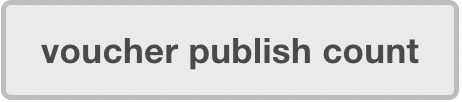`VOUCHER_PUBLISH_COUNT` Voucher start date Returns the voucher's start date.`VOUCHER_START_DATE` Voucher expiration date Returns the voucher's expiration date.`VOUCHER_EXPIRATION_DATE` Promotion tier start date Returns the promotion tier's start date.`PROMOTION_TIER_START_DATE` Promotion tier expiration date Returns the promotion tier's expiration date.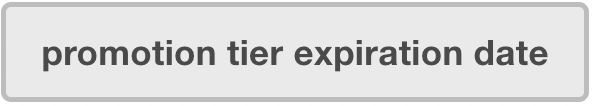`PROMOTION_TIER_EXPIRATION_DATE` Customer birthdate Returns the customer's birthdate.`CUSTOMER_BIRTHDATE` Day of month Returns number of day of month in a date. YYYY-MM- DDVariable Customer's whose birthday falls on the 6th of the month get a 10% off their purchase. All other customers get a 5% off. IF(DAY_OF_MONTH(CUSTOMER_BIRTHDATE) = 6;10;5) Month Returns number of month in a date. YYYY- MM-DDVariable Incentivize customers to make orders through the year. Customer's receive higher amount discounts applied to their order with each consecutive month. The value of the current month is multiplied by a factor of 2. MONTH(TODAY) * 2 Year Returns year in a date. YYYY-MM-DDVariable Incentivize younger folks to make purchases by giving higher amount discounts for younger customers. YEAR(CUSTOMER_BIRTHDATE) / 100 Today Returns today's date. You need to combine this operator with another one, such as Day of month, month or year to extract data from Today. YYYY-MM-DD Variable Give a percentage discount based on the day of the month, i.e. the percent discount will be 11% off on the 11th day of the month. DAY_OF_MONTH(TODAY) Now Returns current date and time, i.e. 2023-03-06T09:04:32.694Z. You need to combine this operator with another one, such as Day of mont, month or year to extract data from Now. YYYY-MM-DDThh:mm:ss.sss Current Take an amount off the order equal to the current month. For March, take \$3 off, for December take \$12 off the order. MONTH(NOW) Date Returns the chosen date, for example 2023-08-17. YYYY-MM-DD Variable Incentivize customers to buy an item on its release date (2023-08-17) to have a \$10 discount. Otherwise, they receive a \$5 discount. IF(DATE("2023-08-17");10;5) Date time Returns the chosen date and time, for example 2023-08-17T14:05:00.000Z. YYYY-MM-DDThh:mm:ss.sss Variable Incentivize customers to buy an item within 5 days since its release date and time to have a \$10 discount. Otherwise, they will receive a \$5 discount. IF(DAYS_SINCE(DATE_TIME("2023-08-17T14:05:00.000Z")) < 5;10;5)

## The Cheapest & Most Expensive Order Item

How are the cheapest and most expensive order items found if there are multiple items with the same price?

Cheapest Order Item Most Expensive Order Item

This is how the cheapest order item is determined.

This is how the most expensive order item is determined.

1
First, the formula tries to find the order item with the cheapest price. If there is one item, the formula returns this item. Otherwise, it proceeds to step 2.
2
If there are  `n` items that have the same cheapest price, from this  `n`, the formula finds the items with the l owest subtotal. If there is only one item, the formula returns this item. Otherwise, if there are many items, it proceeds to step 3.
3
Finally, it returns the first item from the list of the cheapest items with the lowest subtotal.
1
First, the formula tries to find the order item with the most expensive price. If there is one item, the formula returns this item. Otherwise, it proceeds to step 2.
2
If there are  `n` items that have the same most expensive price, from this  `n`, the formula finds the items with the lowest subtotal. If there is only one item, the formula returns this item. Otherwise, if there are many items, it proceeds to step 3.
3
Finally, it returns the first item from the list of the most expensive items with the lowest subtotal.

## Operator reference

### Number Operators

Here are the discount formula operators you can choose from for properties of Number type.

 Operator How does it work? Use case Add + Adds numbers or expressions. Example with missing metadata property defaulting to fallback value `CUSTOMER_METADATA("number_of_store_visits") + REDEEMABLE_METADATA("active_on_calendar_month")` Missing metadata property: number_of_store_visits `Fallback value: 10%` Subtract - Subtracts numbers or expressions. Give a customer a percent off for every year since they first became a customer. `PUBLICATION_METADATA("year") - CUSTOMER_METADATA("customer_life_time_value")`  ⤵ year: 2022 customer_life_time_value: 2012 Calculated dynamic discount value: 10% Fallback value: 10% Multiply x Multiplies numbers or expressions. Give a customer a discount proportional to the day of the week if the week are defined as:  Sunday -         `0` Monday -        `1` Tuesday -       `2` Wednesday -  `3` Thursday -      `4` Friday -           `5` Saturday -       `6` `ORDER_METADATA("day_of_week") x 2`  ⤵ day_of_week: 5 Calculated dynamic discount value: \$10 Fallback value: \$10 Divide ÷ Divides numbers or expressions. Give a customer an eighth for every month the customer was active. `CUSTOMER_METADATA("customer_months_active") ÷ 8`  ⤵ customer_months_active: 8 Calculated dynamic discount value: \$10 Fallback value: \$10 Modulo % Returns the  remainder after division, i.e. returns the amount "left over" after dividing one number or expression by another. Give a customer a certain number of free items based on a custom formula. `REDEEMABLE_METADATA("active_on_calendar_month") % REDEMPTION_METADATA("allowed_free_units")`  ⤵ active_on_calendar_month: 10 allowed_free_units: 9 remainder: 1 Calculated dynamic discount value: 1 Free item Fallback value: 1 Free item Greater than > Logical operator returns the Value if true if the value on the left is greater than the value on the right. Otherwise, it returns the Value if false. Give a customer 20% off every time they make a purchase after their 10 th store visit. Otherwise, the customer receives a standard 3% discount. IF(CUSTOMER_METADATA("number_of_store_visits") > 10;20;3) Less than < Logical operator returns the Value if true if the value on the left is less than the value on the right. Otherwise, it returns the Value if false. Give a customer 3% off each store visit until their 9 th store visit. Then, beginning from their 10th store visit, give the customer a 20% discount.   IF(CUSTOMER_METADATA("number_of_store_visits") < 10;3;20) Equal to = Logical operator returns the  Value if true if the value on the left is equal to the value on the right. Otherwise, it returns the Value if false. Give a customer 50% off on their 50 th store visit. IF(CUSTOMER_METADATA("number_of_store_visits") = 50;50;0) In array Logical operator returns the value true if the value on the left is one of the values provided on the right. Otherwise, it returns false. Give a customer a \$10 discount if the number of units in an order is 3, 6, 9, 12, or 15. Otherwise, they get a 5\$ discount. IF(ORDER_UNITS_QUANTITY IN_ARRAY "3, 6, 9, 12, 15";10;5) Not in array Logical operator returns the value true if the value on the left is NOT one of the values provided on the right. Otherwise, it returns false. Give a customer a \$10 discount if the number of units in an order is NOT 1, 2, 3, or 4. Otherwise, they get a \$10 discount. IF(ORDER_UNITS_QUANTITY NOT_IN_ARRAY "1, 2, 3, 4";10;5)

### String Operators

Here are the discount formula operators you can choose from for properties of String type.

### Date Operators

Here are the discount formula operators you can choose from for properties of Date type.

 Operator How does it work? Use case Days since Returns the number of days from the start date (variable) to the end date (current date), excluding the end date. YYYY-MM- DDCurrent - YYYY-MM-DDVariable # days excluding end date Incentivize customers to make another purchase after they make an order by creating a discount that depreciates with the number of days since they last made a purchase. IF(DAYS_SINCE(CUSTOMER_METADATA ("date_last_purchase")) < 31;30 / DAYS_SINCE(CUSTOMER_METADATA ("date_last_purchase"));0) Months since Returns the number of months from the start date (variable) to the end date (current date) excluding the end date. YYYY- MM-DDCurrent - YYYY-MM-DDVariable # months excluding end date If a customer has been subscribed for more than 12 months, they will get a discount of 20%. Otherwise, they will get 10%.   IF(MONTHS_SINCE(CUSTOMER_METADATA ("date_subscribed")) > 12;20;10) Years since Returns number of years from the start date (variable) to the end date (current date) excluding the end date. YYYY-MM-DDCurrent - YYYY-MM-DDVariable # years excluding end date Give customers an increasing discount based on the number of years they are a customer. YEARS_SINCE(CUSTOMER_METADATA ("acquisition_date")) Get a discount equal to your age! YEARS_SINCE(CUSTOMER_BIRTHDATE) Days until Returns the number of days from the start date (current date) to the end date (variable) excluding the end date. YYYY-MM- DDVariable - YYYY-MM-DDCurrent # days excluding end date Early bird scenario: If you want customers to buy the items sooner, you can influence the discount based on the number of days from/to a specific date.  A decreasing percentage discount that goes to zero at the voucher expiration date. For each day until the expiration date, the customer will get 2 percent off. `DAYS_UNTIL(VOUCHER_EXPIRATION_DATE) * 2` Months until Returns the number of months from the start date (current date) to the end date (variable) excluding the end date. YYYY- MM-DDVariable - YYYY-MM-DDCurrent # months excluding end date Customers can buy six months or more prior to new year's eve and pay 15% less!. Otherwise, they will get 5% off. Here, the expiration date would be set to new year's eve. IF(MONTHS_UNTIL(VOUCHER_EXPIRATION_DATE) > 6;15;5) is Returns true if the date on the left is the same as the date on the right. Otherwise, it returns false. Give a customer a \$10 discount if today is a specific day. Otherwise, they get a \$5 discount. IF(TODAY DATE_IS DATE("2023-08-22");10;5) Is not Returns true if the date on the left is NOT the same as the date on the right. Otherwise, it returns false. Give a customer a \$10 discount if today is NOT a specific day. Otherwise, they get a \$5 discount. IF(TODAY DATE_IS_NOT DATE("2023-08-22");10;5) Is after Returns true if the date on the left is after the date on the right. Otherwise, it returns false. Give a customer a \$10 discount if today is after a specific date. Otherwise, i.e. before the defined date, they get a \$5 discount. IF(TODAY IS_AFTER DATE("2023-08-22");10;5) Is before Returns true if the date on the left is before the date on the right. Otherwise, it returns false. Give a customer a \$10 discount if they place an order before a specific date. Otherwise, i.e. after the defined date, they get a \$5 discount. IF(NOW IS_BEFORE DAY_OF_MONTH(DATE("2023-08-22"));10;5)

### Complex Expression Operators

Here are the discount formula operators you can choose from for creating complex expressions.

 Operand How does it work? Use case And Two or more components can be logically joined using the logical operator `AND`. Note: All components must be true for the complex expression to be true. Give a customer 20% off every time they make a purchase after their 10 th store visit AND the customer makes a purchase on a Friday. Otherwise, the customer receives a standard 3% discount. IF(CUSTOMER_METADATA("number_of_store_visits") > 10 AND ORDER_METADATA("day_of_week") = 5;20;3) Or Two or more components can be logically joined using the logical operator `OR`. Note: If at least one component is true, the complex expression is true. Give a customer 20% off every time they make a purchase after their 10 th store visit OR the customer makes a purchase on a Friday. Otherwise, the customer receives a standard 3% discount. IF(CUSTOMER_METADATA("number_of_store_visits") > 10 OR ORDER_METADATA("day_of_week") = 5;20;3)

### Nested Formulas

You can create nested expressions by embedding statements within true and false blocks of logical IF statements.

 How does it work? Use case If a condition is true, the logical expression will follow the nested path defined inside the condition. If the condition is false, the logical expression will follow the other path. Give a customer 25% off if the order amount is greater than 200 and the quantity of items in the cart is more than 4. Otherwise, give the customer 15% off. If the customer does not have more than 4 items in the cart, the customer gets 10% off. IF(ORDER_ITEMS_QUANTITY > 4;IF(ORDER_AMOUNT > 200;25;15);10)

### Switch Function

You can create expressions using the switch function to create different discount rates based on a given factor. For example, give a different discount rate based on where the order is placed.

 How does it work? Use case Switch the discount value based on a predefined factor. Give a customer 10% off if the order is made in a Boston store, 15% if the order transaction occurs in a New York store. Otherwise, give the customer a 5% discount. SWITCH(ORDER_METADATA("store_city");SWITCH_CASE("Boston";10) SWITCH_CASE("New York";15);5)